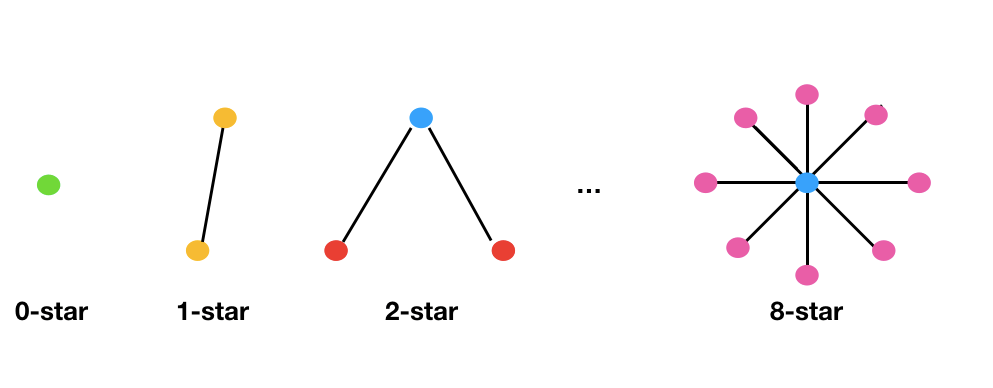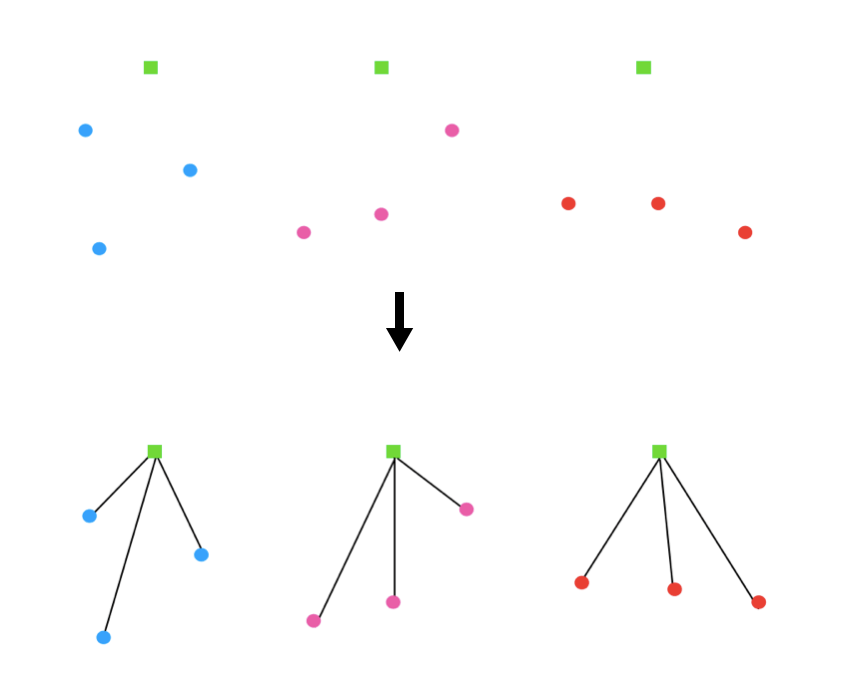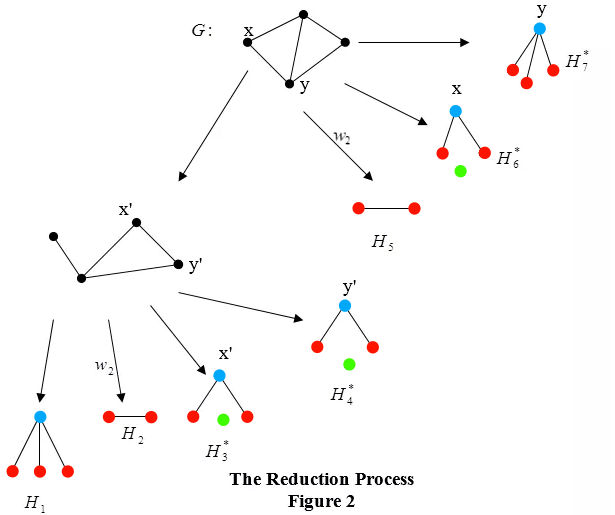# Star polynomials

Natalya Silcott introduces us to star polynomials!This post is part of black mathematician month.Edward J Farrell is a celebrated mathematician of the African Diaspora. In 1978, he introduced a general class of graph polynomials, called Farrell-polynomials. Let $F$ be a family of connected graphs (meaning there exists a path between each pair of nodes – a path can be made up of edges and other nodes). With each element $\alpha$ belonging to $F$, we can associate an indeterminate or weight $w_{\alpha}$. An $F$-cover of a graph $G$ is a spanning subgraph, all of whose components belong to $F$. A spanning subgraph is a subgraph which contains all nodes of the original graph. With each $F$-cover $C$ of $G$, we associate the weight $w(C)=\prod w_{\alpha}$, where the product is taken over all the components $\alpha$ in $C$. The $F$-polynomial of $G$ is $F(G;\underline{w})=\sum w(C)$, where $\underline{w}$ is a vector of indeterminates defined by $w_{\alpha}$ and the summation is taken over all the $F$-covers in $G$.

A tree is a graph in which any pair of nodes is connected by exactly one path. An $m$-star $S_m$ is a tree with $m+1$ nodes, containing a node of valency (degree) $m$ called the centre of the star, meaning it is joined to $m$ other nodes. These $m$ nodes of valency 1 are called tips. A proper star is a star that contains at least one edge. A 0-star is a component node and a 1-star is a component edge as shown below.Now let $F$ be the family of stars and $G$ be a graph with $p$ nodes. A star cover of $G$ is a spanning subgraph of $G$ in which every component is a star. A proper star cover contains no component nodes. A simple $m$-cover of $G$ is a star cover consisting of an $m$-star and $(p-m-1)$ isolated nodes. Throughout this article, the word cover means a star cover, unless otherwise qualified. If we assign to every $m$-star in $G$ a weight $w_{m+1}$, then the weight of a cover $C$ of $G$, with $r$ components $S_{m_1},S_{m_2}, \ldots, S_{m_r}$, will be $w(C)=\prod_{i=1}^r w_{m_i}$. The star polynomial of $G$ is then $E(G;\underline{w})=\sum w(C)$, where the summation is taken over all the covers $C$ of $G$, and $\underline{w}$ is a vector of indeterminates $w_1,w_2,\ldots, w_p$. For brevity, we write $E(G)$ for $E(G;\underline{w})$. If we replace $w_i$ by $w$ for all values of $i$, then the resulting polynomial in $w$ is called the simple star polynomial of $G$ and is denoted by $E(G;w)$.Farrell (1978) introduced star polynomials of various classes of graphs and discussed results about node-disjoint decomposition of complete graphs and complete bipartite graphs. A complete graph is one in which each pair of distinct nodes is connected by an edge. A bipartite graph is one in which the nodes can be decomposed into two sets with every edge only connecting nodes from the two different sets. Explicit formulae, in terms of subgraphs of the graph were derived for the first six coefficients of the simple star polynomial of a graph (Farrell, 1981). From these results, explicit formulae for the number of ways of covering the nodes of the wheel, fan, short ladder, long ladder and lattice, with $k$ stars, for some values of $k$ were deduced.

Farrell and de Matas (2000) derived explicit formulae for the star polynomials of some families of graphs with small cyclomatic number, namely, long stars, tadpoles, figure-8 graphs, dumbbells and theta-graphs. In de Matas (1986), the generating functions for the star polynomials of wheels, fans and graphs with chains added are given. The ordinary generating functions for the star polynomials of chains and cycles and the exponential generating functions for complete graphs and complete bi-partite graphs are given in Farrell (1978). The suspended graph $G+x$ is the graph formed by joining $x$, a component node not in $G$, to every node of $G$. In Farrell and de Matas (2002), a formula was given for the star polynomial of $G+x$. Explicit formulae were given for the suspended chain (fan), the suspended cycle (wheel) and the suspended star. Results on the number of node-disjoint decompositions of these graphs into stars were also deduced.

Star polynomials have the following properties (Farrell, 1978):

The Component theorem:
(i) The coefficient of $w_1^p$ in $E(G;\underline{w})$ is equal to one.
(ii) The coefficient of $w_1^{p-2} w_2$ in $E(G;\underline{w})$ is the number of edges in $G$.
(iii) If $G$ is the null graph (an edgeless graph) , then $E(G;\underline{w})=1$.
(iv) If $G$ is a component node, then $E(G;\underline{w})=w_1$.
(v) If $G$ is an edge, then $E(G;\underline{w})=w_1^2+w_2$.
(vi) If $G$ is non-null, then the constant term in $E(G;\underline{w})$ is zero.
(vii) If $G$ has $k$ components $G_1,G_2,\ldots, G_k$ then $E(G;\underline{w})=\prod_{i=1}^k E(G_i;\underline{w})$

We will now look at one method of finding star polynomials of graphs. Let $G$ be a graph containing an edge $xy$. By the incorporation of an edge $xy$, we mean that it is required to belong to every cover in $G$ that we consider. A restricted graph is a graph containing at least one incorporated edge. The following result was given in Farrell (1978).

Let $G$ be a graph and $\alpha$, an edge in $G$, then $F(G;\underline{w})= F(G’;\underline{w})+(G^*;\underline{w})$, where $G’$ is the graph obtained from $G$ by deleting $\alpha$, and $G^*$ is the restricted graph in which $\alpha$ is incorporated. We can apply this result to the special case in which $F$ is the family of stars. It yields $E(G)=E(G’)+E(G*[\alpha])$, where $G^*[\alpha]$ is the graph in which $\alpha$ is incorporated.

Suppose that $\alpha$ joins nodes $x$ and $y$. $\alpha$ can be incorporated in three ways:
(i) $xy$ is a component star.
(ii) $x$ is the centre of an $m$-star ($m>1$) and $y$ is a tip.
(iii) $y$ is the centre of an $m$-star ($m>1$) and $x$ is a tip.
then, $E(G*[\alpha])= w_2 E(G-xy)+E(G^*[x])+E(G^*[y])$, where $E(G^*[x])$ is the restricted graph in which $x$ is the centre of an $m$-star ($m>1$) and $E(G^*[y])$ is similarly defined.

Thus, we get the following result, also established in Farrell (1978):
$$E(G)=E(G’)+w_2 E(G-xy)+E(G^*[x])+E(G^*[y]).$$
We can find the star polynomial of an arbitrary graph $G$, by applying this result to $G$, until we obtain graphs whose star polynomials could easily be written down. This process is called the Fundamental Edge Algorithm for star polynomials. We will refer to this algorithm as the Reduction Process and it is illustrated below.

Let $G$ be a graph consisting of a quadrilateral with one diagonal. As shown in figure 2, we can apply the reduction process to the graph $G$. Restricted graphs are illustrated with an asterisk.\begin{align}E(H_1) &=w_1^4+3w_1^2 w_2+ 3w_1 w_3 + w_4, \nonumber \\
E(H_2) &=w_1^2 w_2+w_2^2, \nonumber \\
E(H_3^*) &=w_1 w_3, \nonumber \\
E(H_4^*) &=w_1w_3, \nonumber \\
E(H_5) &=w_1^2w_2+w_2^2, \nonumber \\
E(H_6^*) &=w_1w_3,  \nonumber \\
E(H_7^*) &=w_4+2w_1w_3. \end{align}
By summing the contributions of these graphs, we get: $$E(G;\underline{w})=w_1^4+5w_1^2 w_2+8 w_1w_3+2w_2^2+2w_4.$$
Other algorithms for finding star polynomials of graphs are given in Farrell and de Matas (1994). In Farrell and de Matas (1998), it was shown that the partition of a graph can be determined from its star polynomial and an algorithm for doing so was given. The known result that the partition of a graph is reconstructible from the set of node-deleted subgraphs was subsequently shown.

Star polynomials have been useful in the analysis and investigation of a variety of assignment problems. In Farrell (1994), it was shown the star polynomial can be useful in problems involving the optimum location of facilities, general communications networks and computer networks. An $X$-centred star cover of a graph $G$ is a star cover in which the centre of each star belongs to $X$, and all the tips belong to $V(G)-X$, where $X \subseteq V(G)$, the node set of $G$. The $X$-centred star covers can be used for solving set covering problems. These star covers are also useful in the solution of timetabling problems (de Matas, 1999).

Minimum weight star covers can be used to solve location problems for facilities in a network. Algorithms for solving certain types of minimax and minisum location problems can be used to produce minimum weight star covers of an edge weighted graph $G$. Possible applications to coding theory and clustering statistical data are also discussed.

#### References

de Matas, C.M. 1986. On Star Polynomials of Graphs, M. Phil. Thesis, U.W.I. St. Augustine.
de Matas, C.M. 1999. Star Covers of Graphs: Their Polynomials and Applications, Ph. D. Thesis, U.W.I. St. Augustine.
Farrell, E.J. 1978. On a Class of Polynomials associated with the Stars of a Graph and its Application to Node Disjoint Decompositions of Complete Graphs and Complete Bipartite Graphs into Stars, Canadian Math, Bulletin, Vol. 2. 1: 35-46.
Farrell, E.J. 1981. On Coefficients of Star Polynomials, Proc. 3rd. Caribb. Conf. in Combinatorics and Computing, 110-124.
Farrell, E.J. 1994. Star Polynomials: Some Possible Applications, Proc.
Carib. Academy of Sciences, Vol. 5. 151-160.
Farrell, E.J. and de Matas, C.M.1988. On Star Polynomials, Graphical Partitions and Reconstruction, International Journal of Math. and Math. Science,Vol. 11, 1: 87–94.
Farrell, E.J. and de Matas, C.M. 2000. Star Polynomials of Some Families of Graphs with Small Cyclomatic Numbers, Utilitas Mathematica, 58: 87-96.
Farrell, E.J. and de Matas, C.M. 2002. Star Decompositions of Suspended Graphs, Utilitas Mathematica, 61:181-192.Natalya is a teacher at Harrow School and is interested in graph theory
+ More articles by Natalya